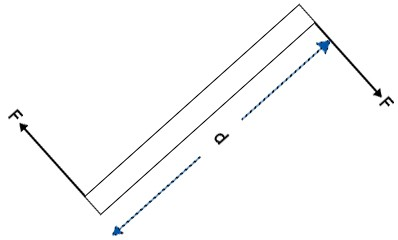### Equation of forces II

Physics SSS2 First Term

Sub Theme: Interaction of matter, space and time

WEEK 7

Equation of forces II

Performance Objectives

Students should be able to;

1. Explain what is meant by the centre of gravity of a body.

2. Identify its position for some regular uniform bodies.

Couple

Content

A couple is a system of equal and opposite forces acting at a distance apart and whose lines of actions do not coincide. A couple will always create a turning effect about a point midway between the two forces.

The forces of a couple create a torque. Couple causes an angular acceleration.The moment of a couple = F × d

The perpendicular distance between the two forces is called the arm of the couple. The moment of a couple about any point in a plane.... View More